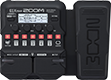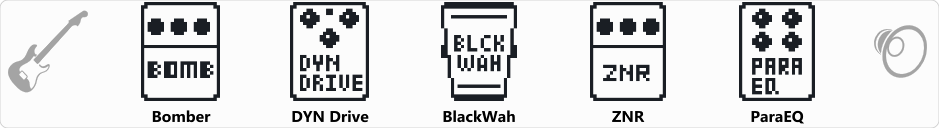Discussion in 'Zoom G1/G1X Four' started by Zacc, Aug 15, 2021.Device: Zoom G1X Four
Firmware: 2.00

Optimized for: Guitar Amp

Effects chain:Put the pedal all the way up if not using the wah part. Distorted radio sounding tone

Effect: "Bomber" (Sfx), active - "yes"
"Decay" = 64
"Tone" = 10
"Mix" = 61
"ON/OFF" = TRGGR

Effect: "DYN Drive" (Overdrive / Distortion), active - "yes"
"Gain" = 100
"Tone" = 100
"Mode" = STACK
"Volume" = 40

Effect: "BlackWah" (Pedal), active - "yes"
"Frequency" = 0
"Range" = 0
"Dry" = 65
"Volume" = 52

Effect: "ZNR" (Dynamics), active - "yes"
"Detect" = EFXIN
"Depth" = 100
"Threshold" = 100
"Decay" = 48

Effect: "ParaEQ" (Filter), active - "yes"
"Frequency" = 125Hz
"Q" = 0.6
"Gain" = 0.0
"Volume" = 80

Patch Volume: 50